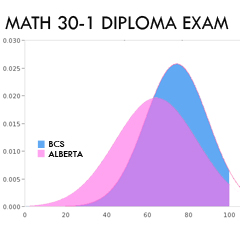Math 30-1 Diploma ExamIn January, my grade 12 math students wrote their diploma exam, a provincial requirement and a university placement helper. They did superbly! I have to brag a little since I had a class of 24 with wide ranging skills and because of a schoolwide scheduling change 15% less instructional time to deliver the course than what was previously allotted (and recommended, and possibly required…).

The course is very demanding with nine pre-calculus units:

• Functions
and
Relations

• Transformations on Functions
• Exponential
and
Logarithmic
Functions
• Applications
of
Exponential
and

Logarithmic
Functions
• Polynomial
Functions
and
Equations
• Permutations
and
Combinations
• Analyzing
and
Rational
Functions
• Trigonometry
–
Functions
and
Graphs
• Trigonometry
–
Equations
and
Identities
My students scored on average 10% higher than the province (which had a 24% failure rate). We also had a tight standard deviation of 15.5 whereas the province had a spread of 20.1.
So! Congratulations to my stellar students!

1.2.Bill